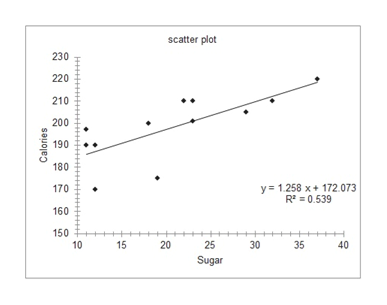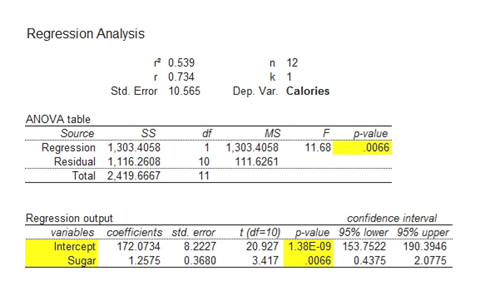# You have been asked to find if there is a correlation between the amount of calories per serving in a bowl of cereal versus the amount of sugar (in grams) in the cereal.  The following data has been given for examination. Sugar Calories 182002321012170121903722019175232012221032210111901119729205a.    Create a scatterplot of the given data.  Be sure you explain your decision on which variable is the predictor and which variable is the response.  b.    Using computer software, calculate the correlation coefficient and interpret what this value implies in relation to the data set. c.    Create a regression equation that models the data and describe the values of the slope and y-intercept with the data set. d.    Predict if a box of cereal has 25 grams of sugar per serving how many calories it will contain. e.    Predict if a box of cereal has 230 calories per serving how much sugar (in grams) it will contain. f.    Discuss any lurking variables could be relevant to analysis of this data set.

Question
28 views

You have been asked to find if there is a correlation between the amount of calories per serving in a bowl of cereal versus the amount of sugar (in grams) in the cereal.  The following data has been given for examination.

 Sugar Calories 18 200 23 210 12 170 12 190 37 220 19 175 23 201 22 210 32 210 11 190 11 197 29 205

a.    Create a scatterplot of the given data.  Be sure you explain your decision on which variable is the predictor and which variable is the response.
b.    Using computer software, calculate the correlation coefficient and interpret what this value implies in relation to the data set.
c.    Create a regression equation that models the data and describe the values of the slope and y-intercept with the data set.
d.    Predict if a box of cereal has 25 grams of sugar per serving how many calories it will contain.
e.    Predict if a box of cereal has 230 calories per serving how much sugar (in grams) it will contain.
f.    Discuss any lurking variables could be relevant to analysis of this data set.

check_circle

Step 1

Note:

Hey there! Thank you for posting the question. However, since your question has more than 3 parts, according to our policy, we have solved the first 3 parts for you. If you need help with any other part, please re-post the question and mention the part you need help with.

Step 2

a.

Step-by-step procedure to obtain a scatter plot using Excel add-in Mega stat as follows:

• Enter the data into Excel spreadsheet.
• Choose Mega Stat > Correlation/Regression > Scatterplot.
• In Input ranges, enter the values of horizontal axis, Vertical axis, and chart title.
• Check the appropriate options in Display.
• Click OK.

The output obtained using Excel add-in Mega stat is represented as follows:

From the above graph, the correlation between the sugar (Predictor) and Calories (Response) are positive.

As grams of sugar increases in the box of cereals then Calories also increase in the same direction.help_outlineImage Transcriptionclosescatter plot 230 220 210 200 190 180 y 1.258 x+172.073 R2-0.539 170 160 150 10 15 35 25 Sugar 40 30 20 Calories fullscreen
Step 3

b.

The correlation coefficient can be obtained as follows:

Step-by-step procedure to obtain the correlation Excel add-in Mega stat as follows:

• Choose Mega Stat > Correlation/Regression > Regression.
• In Input ranges, enter the values of Sugar in independent variable.
• In Input ranges, enter the values of Calories in dependent variable
• Check the appropriate options in Display.
• Click OK.

The output obtained using Exc...help_outlineImage TranscriptioncloseRegression Analysis 0.539 n 12 k 1 r 0.734 Std. Error 10.565 Dep. Var. Calories ANOVA table Source Regression 1,303.4058 Residual 1,116.2608 Total SS MS p-value 0066 F df 1 1,303.4058 11.68 10 11 111.6261 2,419.6667 Regression output vaniables coefficients std. error Intercept Sugar confidence interval t (df=10) p-value 95% lower 95% upper 20.927 1.38E-09 153.7522 3.417 172.0734 8.2227 190.3946 1.2575 0.3680 0066 0.4375 2.0775 fullscreen

### Want to see the full answer?

See Solution

#### Want to see this answer and more?

Solutions are written by subject experts who are available 24/7. Questions are typically answered within 1 hour.*

See Solution
*Response times may vary by subject and question.
Tagged in

### Other